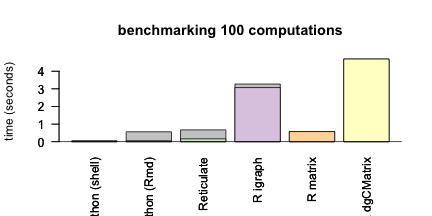knitr::opts_chunk\$set(
collapse = TRUE,
comment = "#>",
eval = module
)
print(module)
#>  TRUE

Benchmarking the Leiden Algorithm

In this guide we will run the Leiden algorithm in both R and Python to benchmark performance and demonstrate how the algorithm is called with reticulate.

We are testing this in the following environment:

paste(Sys.info()[c(4, 2, 1)])
#>  "MacBook-Pro" "19.6.0"      "Darwin"
R.version\$version.string
#>  "R version 4.1.0 (2021-05-18)"

Clustering with the Leiden Algorithm in R

This package allows calling the Leiden algorithm for clustering on an igraph object from R. See the Python and Java implementations for more details:

https://github.com/CWTSLeiden/networkanalysis

https://github.com/vtraag/leidenalg

It calls the Python functions to run the algorithm and passes all arguments need to them.

Python implementation

The python version can be installed with pip or conda:

pip uninstall -y igraph
pip install -U -q leidenalg
conda install -c vtraag leidenalg

It is also possible to install the python dependencies with reticulate in R.

library("reticulate")
py_install("python-igraph")
py_install("leidenalg")

Running in Python

We are using the following version of Python:

import sys
print(sys.version)
#> 3.8.6 | packaged by conda-forge | (default, Oct  7 2020, 18:49:01)
#> [Clang 10.0.1 ]

import igraph as ig
print("igraph", ig.__version__)
#> igraph 0.8.3
import leidenalg as la
print("leidenalg", la.__version__)
#> leidenalg 0.8.3

Then we load the Zachary karate club example data from igraph.

G = ig.Graph.Famous('Zachary')
G.summary()
#> 'IGRAPH U--- 34 78 -- '
partition = la.find_partition(G, la.ModularityVertexPartition)
print(partition)
#> Clustering with 34 elements and 4 clusters
#>  8, 9, 14, 15, 18, 20, 22, 26, 29, 30, 32, 33
#>  0, 1, 2, 3, 7, 11, 12, 13, 17, 19, 21
#>  23, 24, 25, 27, 28, 31
#>  4, 5, 6, 10, 16
partition
#> <leidenalg.VertexPartition.ModularityVertexPartition object at 0x7f9de8fbd580>
partition.membership
#> [1, 1, 1, 1, 3, 3, 3, 1, 0, 0, 3, 1, 1, 1, 0, 0, 3, 1, 0, 1, 0, 1, 0, 2, 2, 2, 0, 2, 2, 0, 0, 2, 0, 0]
partition <- py\$partition\$membership + 1
table(partition)
#> partition
#>  1  2  3  4
#> 12 11  6  5

We can plot the result in R to show it in the network. This reproduces the example in the Python leidenalg documentation.

library("igraph")
#>
#> Attaching package: 'igraph'
#> The following objects are masked from 'package:stats':
#>
#>     decompose, spectrum
#> The following object is masked from 'package:base':
#>
#>     union
library("reticulate")
library("RColorBrewer")
graph_object <- graph.famous("Zachary")
node.cols <- brewer.pal(max(c(3, partition)),"Pastel1")[partition]
plot(graph_object, vertex.color = node.cols, layout=layout_with_kk)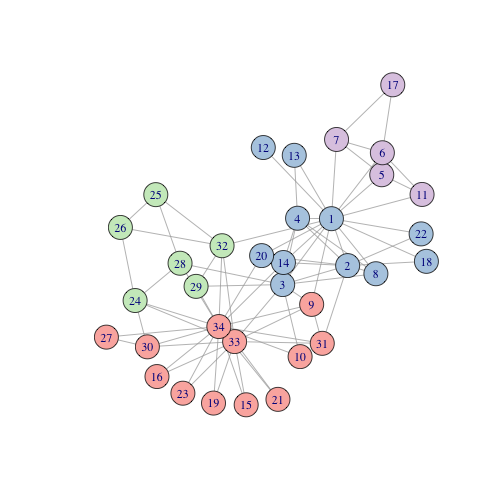We can reproduce passing arguments in this manner as well.

partition = la.find_partition(G, la.CPMVertexPartition, resolution_parameter = 0.05)
print(partition)
#> Clustering with 34 elements and 2 clusters
#>  8, 14, 15, 18, 20, 22, 23, 24, 25, 26, 27, 28, 29, 30, 31, 32, 33
#>  0, 1, 2, 3, 4, 5, 6, 7, 9, 10, 11, 12, 13, 16, 17, 19, 21
partition
#> <leidenalg.VertexPartition.CPMVertexPartition object at 0x7f9de8fbdd30>
partition.membership
#> [1, 1, 1, 1, 1, 1, 1, 1, 0, 1, 1, 1, 1, 1, 0, 0, 1, 1, 0, 1, 0, 1, 0, 0, 0, 0, 0, 0, 0, 0, 0, 0, 0, 0]
partition <- py\$partition\$membership + 1
table(partition)
#> partition
#>  1  2
#> 17 17

We can plot the result in R to show it in the network. This reproduces the example in the Python leidenalg documentation.

graph_object <- graph.famous("Zachary")
node.cols <- brewer.pal(max(c(3, partition)),"Pastel1")[partition]
plot(graph_object, vertex.color = node.cols, layout=layout_with_kk)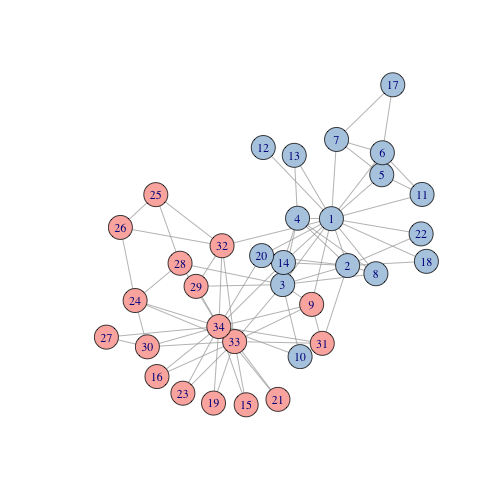We can run the RBC vertex method which generalises the modularity vertex partition.

partition = la.find_partition(G, la.RBConfigurationVertexPartition, resolution_parameter = 1.5)
print(partition)
#> Clustering with 34 elements and 5 clusters
#>  8, 14, 15, 18, 20, 22, 26, 29, 30, 32, 33
#>  0, 1, 3, 7, 11, 12, 13, 17, 19, 21
#>  4, 5, 6, 10, 16
#>  23, 24, 25, 27, 31
#>  2, 9, 28
partition
#> <leidenalg.VertexPartition.RBConfigurationVertexPartition object at 0x7f9de8fc33d0>
partition.membership
#> [1, 1, 4, 1, 2, 2, 2, 1, 0, 4, 2, 1, 1, 1, 0, 0, 2, 1, 0, 1, 0, 1, 0, 3, 3, 3, 0, 3, 4, 0, 0, 3, 0, 0]
partition <- py\$partition\$membership + 1
table(partition)
#> partition
#>  1  2  3  4  5
#> 11 10  5  5  3

We can plot the result in R to show it in the network.

graph_object <- graph.famous("Zachary")
node.cols <- brewer.pal(max(c(3, partition)),"Pastel1")[partition]
plot(graph_object, vertex.color = node.cols, layout=layout_with_kk)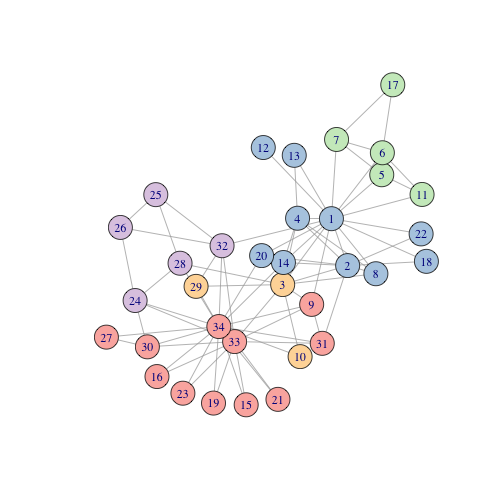Benchmarking the Python version with reticulate

Now we can time how long the computation of the algorithm takes (for 1000 runs) running within python:

import time
G = ig.Graph.Famous('Zachary')
G.summary()
#> 'IGRAPH U--- 34 78 -- '
start = time.time()
for ii in range(100):
partition = la.find_partition(G, la.ModularityVertexPartition)

end = time.time()
partition.membership
#> [1, 1, 1, 1, 3, 3, 3, 1, 0, 0, 3, 1, 1, 1, 0, 0, 3, 1, 0, 1, 0, 1, 0, 2, 2, 2, 0, 2, 2, 0, 0, 2, 0, 0]
py_time = end - start
print("leiden time:", py_time, "seconds")
#> leiden time: 0.055066823959350586 seconds
bash_py_time=`python -c 'import igraph as ig
import leidenalg as la
import time
G = ig.Graph.Famous("Zachary")
G.summary()
start = time.time()
for ii in range(100):
partition = la.find_partition(G, la.ModularityVertexPartition)

end = time.time()
partition.membership
py_time = end - start
print(py_time)'`
echo \$bash_py_time > bash_py_time
echo "leiden time:" \$bash_py_time "seconds"
#> leiden time: 0.04465913772583008 seconds

We can also run the leiden algorithm in python by calling functions with reticulate:

leidenalg <- import("leidenalg", delay_load = TRUE)
ig <- import("igraph", delay_load = TRUE)
G = ig\$Graph\$Famous('Zachary')
G\$summary()
#>  "IGRAPH U--- 34 78 -- "
partition = leidenalg\$find_partition(G, leidenalg\$ModularityVertexPartition)
partition\$membership
#>   1 1 1 1 2 2 2 1 0 1 2 1 1 1 0 0 2 1 0 1 0 1 0 3 3 3 0 3 0 0 0 3 0 0
leidenalg <- import("leidenalg", delay_load = TRUE)
ig <- import("igraph", delay_load = TRUE)
G = ig\$Graph\$Famous('Zachary')
G\$summary()
#>  "IGRAPH U--- 34 78 -- "
start <- Sys.time()
for(ii in 1:100){
partition = leidenalg\$find_partition(G, leidenalg\$ModularityVertexPartition)
}
end <- Sys.time()
partition\$membership
#>   1 1 1 1 3 3 3 1 0 0 3 1 1 1 0 0 3 1 0 1 0 1 0 2 2 2 0 2 2 0 0 2 0 0
reticulate_time <- difftime(end, start)[]
print(paste(c("leiden time:", reticulate_time, "seconds"), collapse = " "))
#>  "leiden time: 0.164825916290283 seconds"

R implementation

The R version can be installed with devtools or from CRAN:

install.packages("leiden")
install.packages("leiden")

Note that these require the Python version as a dependency.

Running in R

We can reproduce these by running the Leiden algorithm in R using the functions in the leiden package.

We are using the following version of R:

R.version.string
#>  "R version 4.1.0 (2021-05-18)"

library("igraph")
library("leiden")

Then we load the Zachary karate club example data from igraph.

G <- graph.famous("Zachary")
summary(G)
#> IGRAPH f4984a5 U--- 34 78 -- Zachary
#> + attr: name (g/c)
partition <- leiden(G, "ModularityVertexPartition")
partition
#>   2 2 2 2 3 3 3 2 1 2 3 2 2 2 1 1 3 2 1 2 1 2 1 1 4 4 1 4 4 1 1 4 1 1
table(partition)
#> partition
#>  1  2  3  4
#> 12 12  5  5

We can plot the result in R to show it in the network. This reproduces the example in the Python leidenalg documentation.

library("igraph")
library("reticulate")
library("RColorBrewer")
node.cols <- brewer.pal(max(c(3, partition)),"Pastel1")[partition]
plot(G, vertex.color = node.cols, layout=layout_with_kk)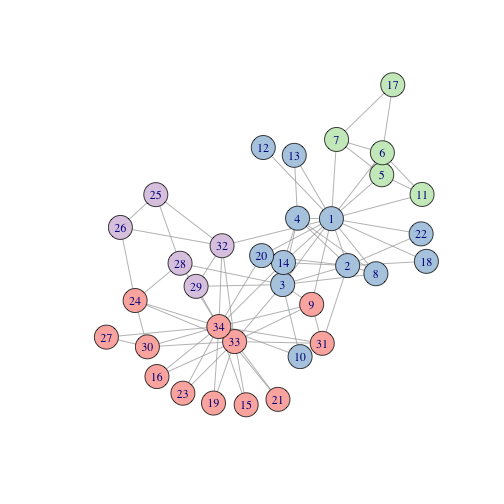We can reproduce passing arguments in this manner as well.

partition <- leiden(G, "CPMVertexPartition", resolution_parameter = 0.5)
partition
#>    1  1  1  1  5  4  4  1  6 10  5 11  9  1 12 19  4 13 14  8 15 16 17  2  3
#>   3 20 18  7  2  6  3  2  2
table(partition)
#> partition
#>  1  2  3  4  5  6  7  8  9 10 11 12 13 14 15 16 17 18 19 20
#>  6  4  3  3  2  2  1  1  1  1  1  1  1  1  1  1  1  1  1  1

We can plot the result in R to show it in the network. This reproduces the example in the Python leidenalg documentation.

node.cols <- brewer.pal(max(c(3, partition)),"Pastel1")[partition]
#> Warning in brewer.pal(max(c(3, partition)), "Pastel1"): n too large, allowed maximum for palette Pastel1 is 9
#> Returning the palette you asked for with that many colors
plot(G, vertex.color = node.cols, layout=layout_with_kk)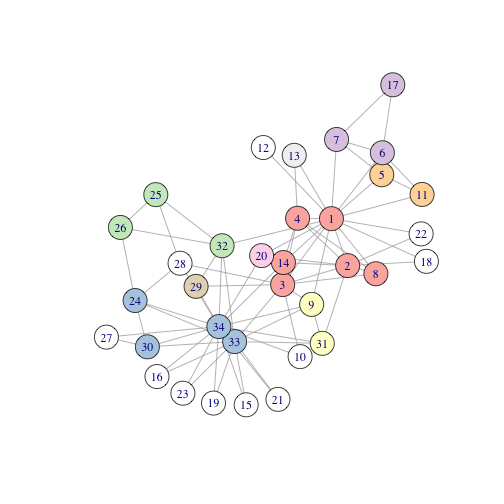We can run the RBC vertex method which generalises the modularity vertex partition.

partition <- leiden(G, "RBConfigurationVertexPartition", resolution_parameter = 1.5)
partition
#>   2 2 5 2 4 4 4 2 1 5 4 2 2 2 1 1 4 2 1 2 1 2 1 3 3 3 1 3 3 1 1 3 1 1
table(partition)
#> partition
#>  1  2  3  4  5
#> 11 10  6  5  2

We can plot the result in R to show it in the network.

node.cols <- brewer.pal(max(c(3, partition)),"Pastel1")[partition]
plot(G, vertex.color = node.cols, layout=layout_with_kk)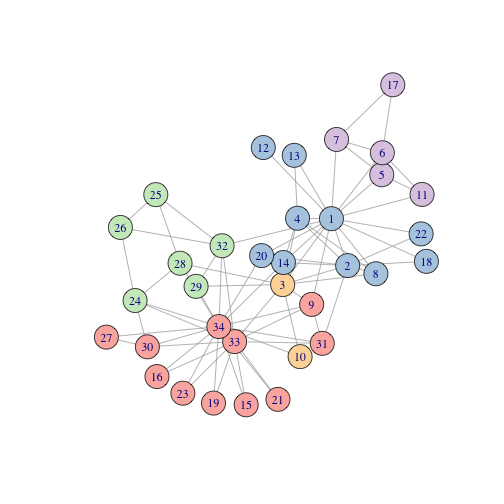Benchmarking the R version with reticulate

Now we can time how long the computation of the algorithm takes (for 1000 runs) calling with R on a graph object:

G <- graph.famous('Zachary')
summary(G)
#> IGRAPH e436d36 U--- 34 78 -- Zachary
#> + attr: name (g/c)
start <- Sys.time()
for(ii in 1:100){
partition = leiden(G, "ModularityVertexPartition")
}
end <- Sys.time()
table(partition)
#> partition
#>  1  2  3  4
#> 12 11  6  5
R_graph_time = difftime(end, start)[]
print(paste(c("leiden time:", R_graph_time, "seconds"), collapse = " "))
#>  "leiden time: 3.07277178764343 seconds"

We can see that the R implementation does not perform as well as the Python version but it is convenient for R users. Calling from a graph object avoids casting to a dense adjacency matrix which reduces memory load for large graph objects.

We can see that calling leiden in R on an adjacency matrix has faster performance but it does require more memory. For example, on a dense adjacency matrix:

G <- graph.famous('Zachary')
summary(G)
#> IGRAPH 6b728fe U--- 34 78 -- Zachary
#> + attr: name (g/c)

start <- Sys.time()
for(ii in 1:100){
}
end <- Sys.time()
#>  34 34
R_mat_cast_time = difftime(end, start)[]
paste(print(c("cast time:", R_mat_cast_time, "seconds"), collapse = " "))
#>  "cast time:"         "0.0112428665161133" "seconds"
#>  "cast time:"         "0.0112428665161133" "seconds"

start <- Sys.time()
for(ii in 1:100){
}
end <- Sys.time()
table(partition)
#> partition
#>  1  2  3  4
#> 12 11  6  5
R_mat_time = difftime(end, start)[]
print(paste(c("leiden time:", R_mat_time, "seconds"), collapse = " "))
#>  "leiden time: 0.576150894165039 seconds"

For example, on a sparse dgCMatrix for the adjacency matrix:

G <- graph.famous('Zachary')
summary(G)
#> IGRAPH 50c8f4a U--- 34 78 -- Zachary
#> + attr: name (g/c)

start <- Sys.time()
for(ii in 1:100){
}
end <- Sys.time()
#>  "dgCMatrix"
#> attr(,"package")
#>  "Matrix"
#>  34 34
R_sparse_mat_cast_time = difftime(end, start)[]
paste(print(c("cast time:", R_sparse_mat_cast_time, "seconds"), collapse = " "))
#>  "cast time:"       "0.13519811630249" "seconds"
#>  "cast time:"       "0.13519811630249" "seconds"

start <- Sys.time()
for(ii in 1:100){
}
end <- Sys.time()
table(partition)
#> partition
#>  1  2  3  4
#> 12 11  6  5
R_sparse_mat_time = difftime(end, start)[]
print(paste(c("leiden time:", R_mat_time, "seconds"), collapse = " "))
#>  "leiden time: 0.576150894165039 seconds"

The difference between sparse and dense matrices is more pronounced for large matrices (with few edges):

adjacency_matrix <- rbind(cbind(matrix(round(rbinom(1000000, 1, 0.008)), 1000, 1000),
matrix(round(rbinom(1000000, 1, 0.003)), 1000, 1000),
matrix(round(rbinom(1000000, 1, 0.001)), 1000, 1000)),
cbind(matrix(round(rbinom(1000000, 1, 0.003)), 1000, 1000),
matrix(round(rbinom(1000000, 1, 0.008)), 1000, 1000),
matrix(round(rbinom(0000000, 1, 0.002)), 1000, 1000)),
cbind(matrix(round(rbinom(1000000, 1, 0.003)), 1000, 1000),
matrix(round(rbinom(1000000, 1, 0.001)), 1000, 1000),
matrix(round(rbinom(1000000, 1, 0.009)), 1000, 1000)))

start <- Sys.time()
for(ii in 1:10){
}
end <- Sys.time()
#>  "matrix" "array"
#>  3000 3000
R_mat_large_cast_time = difftime(end, start)[]
paste(print(c("cast time:", R_mat_large_cast_time, "seconds"), collapse = " "))
#>  "cast time:"        "0.604593992233276" "seconds"
#>  "cast time:"        "0.604593992233276" "seconds"

start <- Sys.time()
for(ii in 1:10){
}
end <- Sys.time()
table(partition)
#> partition
#>    1    2    3
#> 1137 1011  852
R_mat_large_time = difftime(end, start)[]
print(paste(c("leiden time:", R_mat_large_time, "seconds"), collapse = " "))
#>  "leiden time: 21.1848509311676 seconds"

For example, on a sparse adjacency matrix:

start <- Sys.time()
for(ii in 1:100){
}
end <- Sys.time()
#>  "dgCMatrix"
#> attr(,"package")
#>  "Matrix"
#>  3000 3000
R_mat_large_cast_time = difftime(end, start)[]
paste(print(c("cast time:", R_mat_large_cast_time, "seconds"), collapse = " "))
#>  "cast time:"        "0.775704145431519" "seconds"
#>  "cast time:"        "0.775704145431519" "seconds"

start <- Sys.time()
for(ii in 1:10){
}
end <- Sys.time()
table(partition)
#> partition
#>    1    2    3
#> 1052 1013  935
R_mat_large_time = difftime(end, start)[]
print(paste(c("leiden time:", R_mat_large_time, "seconds"), collapse = " "))
#>  "leiden time: 2.09511330127716 seconds"

We compare the processing of adjaceny matrices in the leiden.matrix method to casting to graph in python with reticulate. The current implementation of the R function works as follows. The adjacency matrix is passed to python and the graph object is create in the python-igraph:

partition_type <- "RBConfigurationVertexPartition"
initial_membership <- NULL
weights <- NULL
node_sizes = NULL
resolution_parameter = 1

G <- graph.famous('Zachary')
summary(G)
#> IGRAPH 0b68938 U--- 34 78 -- Zachary
#> + attr: name (g/c)
time1 <- Sys.time()
time2 <- Sys.time()
timing = difftime(time2, time1)[]
print(paste(c("cast to adjacent:", timing, "seconds"), collapse = " "))
#>  "cast to adjacent: 0.00320601463317871 seconds"

#run matrix method
leidenalg <- import("leidenalg", delay_load = TRUE)
ig <- import("igraph", delay_load = TRUE)

#convert matrix input (corrects for sparse matrix input)
} else{
}

#compute weights if non-binary adjacency matrix given
#assign weights to edges (without dependancy on igraph)
weights <- t_mat[t_mat!=0]
#remove zeroes from rows of matrix and return vector of length edges
}

time3 <- Sys.time()
##convert to python numpy.ndarray, then a list
time4 <- Sys.time()
timing = difftime(time4, time3)[]
print(paste(c("pass to python matrix:", timing, "seconds"), collapse = " "))
#>  "pass to python matrix: 0.00466799736022949 seconds"

#convert graph structure to a Python compatible object
GraphClass <- if (!is.null(weights) && !is_pure_adj){
} else {
}
time5 <- Sys.time()
time6 <- Sys.time()
timing = difftime(time6, time5)[]
reticulate_create_time = difftime(time6, time5)[]
print(paste(c("generate graph in python:", timing, "seconds"), collapse = " "))
#>  "generate graph in python: 0.0050809383392334 seconds"

# test performance for computing matrix to graph in R
# other option is to passing snn_graph to Python

time7 <- Sys.time()
#compute partitions
source("../R/find_partition.R")

partition <- find_partition(snn_graph, partition_type = partition_type,
initial_membership = initial_membership ,
weights = weights,
node_sizes = node_sizes,
resolution_parameter = resolution_parameter
)
time8 <- Sys.time()
timing = difftime(time8, time7)[]
print(paste(c("compute partitions:", timing, "seconds"), collapse = " "))
#>  "compute partitions: 0.0514631271362305 seconds"
timing = difftime(time8, time1)[]
print(paste(c("total:", timing, "seconds"), collapse = " "))
#>  "total: 0.0871140956878662 seconds"
partition
#>   2 2 2 2 4 4 4 2 1 1 4 2 2 2 1 1 4 2 1 2 1 2 1 3 3 3 1 3 3 1 1 3 1 1

Is it more efficent to pass to create a graph object in R and pass this to python?

partition_type <- "RBConfigurationVertexPartition"
initial_membership <- NULL
weights <- NULL
node_sizes = NULL
resolution_parameter = 1

G <- graph.famous('Zachary')
summary(G)
#> IGRAPH 743731a U--- 34 78 -- Zachary
#> + attr: name (g/c)
time1 <- Sys.time()
time2 <- Sys.time()
timing = difftime(time2, time1)[]
print(paste(c("cast to adjacent:", timing, "seconds"), collapse = " "))
#>  "cast to adjacent: 0.00363302230834961 seconds"

#run matrix method
leidenalg <- import("leidenalg", delay_load = TRUE)
ig <- import("igraph", delay_load = TRUE)

time3 <- Sys.time()
##convert to python numpy.ndarray, then a list
time4 <- Sys.time()
timing = difftime(time4, time3)[]
print(paste(c("generate graph in R:", timing, "seconds"), collapse = " "))
#>  "generate graph in R: 0.00243210792541504 seconds"

#convert graph structure to a Python compatible object
time5 <- Sys.time()
##convert to list for python input
if(!is.named(object)){
vertices <- as.list(as.character(V(object)))
} else {
vertices <- as.list(names(V(object)))
}

edges <- as_edgelist(object)
dim(edges)
#>  156   2
edgelist <- list(rep(NA, nrow(edges)))
for(ii in 1:nrow(edges)){
edgelist[[ii]] <- as.character(edges[ii,])
}

snn_graph <- ig\$Graph()
time6 <- Sys.time()
timing = difftime(time6, time5)[]
print(paste(c("pass to python graph:", timing, "seconds"), collapse = " "))
#>  "pass to python graph: 0.0667150020599365 seconds"

# test performance for computing matrix to graph in R
# other option is to passing snn_graph to Python

time7 <- Sys.time()
#compute partitions
partition <- find_partition(snn_graph, partition_type = partition_type,
initial_membership = initial_membership ,
weights = weights,
node_sizes = node_sizes,
resolution_parameter = resolution_parameter
)
time8 <- Sys.time()
timing = difftime(time8, time7)[]
print(paste(c("compute partitions:", timing, "seconds"), collapse = " "))
#>  "compute partitions: 0.00444388389587402 seconds"
timing = difftime(time8, time1)[]
print(paste(c("total:", timing, "seconds"), collapse = " "))
#>  "total: 0.0894429683685303 seconds"
partition
#>   2 2 2 2 4 4 4 2 1 1 4 2 2 2 1 1 4 2 1 2 1 2 1 3 3 3 1 3 3 1 1 3 1 1

Another approach is to generate a graph in R and pass it to the leiden.igraph method.

partition_type <- "RBConfigurationVertexPartition"
initial_membership <- NULL
weights <- NULL
node_sizes = NULL
resolution_parameter = 1

G <- graph.famous('Zachary')
summary(G)
#> IGRAPH a65e896 U--- 34 78 -- Zachary
#> + attr: name (g/c)
time1 <- Sys.time()
time2 <- Sys.time()
timing = difftime(time2, time1)[]
print(paste(c("cast to adjacent:", timing, "seconds"), collapse = " "))
#>  "cast to adjacent: 0.00308108329772949 seconds"

time3 <- Sys.time()
##convert to python numpy.ndarray, then a list
time4 <- Sys.time()
timing = difftime(time4, time3)[]
R_graph_create_time = difftime(time4, time3)[]
print(paste(c("generate graph in R:", timing, "seconds"), collapse = " "))
#>  "generate graph in R: 0.00199198722839355 seconds"

#convert graph structure to a Python compatible object
time5 <- Sys.time()
##convert to list for python input
snn_graph <- object
time6 <- Sys.time()
timing = difftime(time6, time5)[]
print(paste(c("pass to R graph:", timing, "seconds"), collapse = " "))
#>  "pass to R graph: 0.00166201591491699 seconds"

# test performance for computing matrix to graph in R
# other option is to passing snn_graph to Python

time7 <- Sys.time()
#compute partitions
partition <- leiden(snn_graph, partition_type = partition_type,
initial_membership = initial_membership ,
weights = weights,
node_sizes = node_sizes,
resolution_parameter = resolution_parameter
)
time8 <- Sys.time()
timing = difftime(time8, time7)[]
print(paste(c("compute partitions:", timing, "seconds"), collapse = " "))
#>  "compute partitions: 0.0360591411590576 seconds"
timing = difftime(time8, time1)[]
print(paste(c("total:", timing, "seconds"), collapse = " "))
#>  "total: 0.0520191192626953 seconds"
partition
#>   2 2 2 2 4 4 4 2 1 1 4 2 2 2 1 1 4 2 1 2 1 2 1 3 3 3 1 3 3 1 1 3 1 1

Here we can see that the current approach to pass adjacency matrices to Python and generate graphs in Python is more efficient for a dense matrix than computing the graph in R. Therefore the leiden.matrix method will not call the leiden.igraph method and they will remain distinct.

Summary

Here we compare the compute time for the Zachary datasets between each method for computing paritions from the leiden clustering algorithm in R or Python.

barplot(c(bash_py_time, py\$py_time, reticulate_time, R_graph_time,
R_mat_time, R_sparse_mat_time),
names = c("Python (shell)", "Python (Rmd)", "Reticulate", "R igraph",
"R matrix","R dgCMatrix"),
col = brewer.pal(9,"Pastel1"), las = 2, srt = 45,
ylab = "time (seconds)", main = "benchmarking 100 computations")
abline(h=0)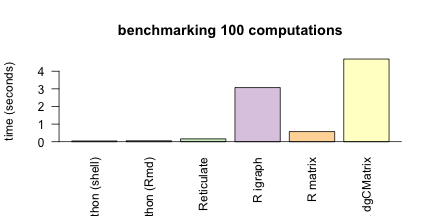If we account for time to cast matrices from graph objects. Then these are the time taken to compute partitions from a graph in R.

barplot(c(bash_py_time, py\$py_time, reticulate_time, R_graph_time, R_mat_time+R_mat_cast_time,
R_sparse_mat_time+R_sparse_mat_cast_time),
names = c("Python (shell)", "Python (Rmd)", "Reticulate", "R igraph",
"R matrix","R dgCMatrix"),
col = "grey80", las = 2, srt = 45,
ylab = "time (seconds)", main = "benchmarking 100 computations")
barplot(c(bash_py_time, py\$py_time, reticulate_time, R_graph_time,
R_mat_time,  R_sparse_mat_time),
names = c("Python (shell)", "Python (Rmd)", "Reticulate", "R igraph",
"R matrix","R dgCMatrix"),
col = brewer.pal(9,"Pastel1"), las = 2, srt = 45,
ylab = "time (seconds)", main = "benchmarking 100 computations", add = TRUE)
abline(h=0)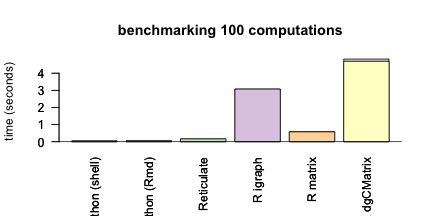Similarly, if we account for time to generate graph from an adjaceny matrix. Then these are the time taken to compute partitions from a matrix in R.

R_graph_create_time = difftime(time4, time3)[]
barplot(c(bash_py_time, py\$py_time+reticulate_create_time*100, reticulate_time+reticulate_create_time*100, R_graph_time+R_graph_create_time*100, R_mat_time,
R_sparse_mat_time),
names = c("Python (shell)", "Python (Rmd)", "Reticulate", "R igraph",
"R matrix","R dgCMatrix"),
col = "grey80", las = 2, srt = 45,
ylab = "time (seconds)", main = "benchmarking 100 computations")
barplot(c(bash_py_time, py\$py_time, reticulate_time, R_graph_time,
R_mat_time,  R_sparse_mat_time),
names = c("Python (shell)", "Python (Rmd)", "Reticulate", "R igraph",
"R matrix","R dgCMatrix"),
col = brewer.pal(9,"Pastel1"), las = 2, srt = 45,
ylab = "time (seconds)", main = "benchmarking 100 computations", add = TRUE)
abline(h=0)